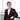# Testing with Mocha: Array Comparison

December 22, 2014

The problem

I was writing a mocha test for an array comparison. Here is the test suite:

describe(‘Array comparison’, function () { ‘use strict’; it(‘should return true if two arrays have the same values’, function () { var myArray = [ ‘a’, ‘b’, ‘c’ ]; expect(myArray).to.equal([ ‘a’, ‘b’, ‘c’ ]); }); });

However, out of my expectation, this test would fail:

AssertionError: expected [ ‘a’, ‘b’, ‘c’ ] to equal [ ‘a’, ‘b’, ‘c’ ]

My Explanation

Why do arrays not compare the way other values do? Because typeof array is an object. In mocha, to.equal does not signify that the operands are semantically equal, but they refer to the exact same object. In other words, the above test fails since myArray is not the exact same object as [ ‘a’, ‘b’, ‘c’ ];

Possible Solutions

1. Use .eql for ‘lose equality’ in order to deeply compare values.
2. Use .deep.equal, which does not test whether the operands are the same object, but rather that they are equivalent.
3. Check .members in the array instead
4. Convert the array to string and compare it.

References

Originally published at victorleungtw.com on November 28, 2014.Written by Victor Leung who is a keen traveller to see every country in the world, passionate about cutting edge technologies. Follow me on Twitter